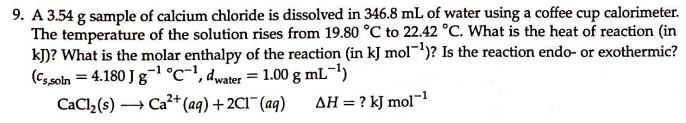# 9. A 3.54 g sample of calcium chloride is dissolved in 346.8 mL of water using a coffee cup calorimeter. The temperature of the solution rises from 19.80 degrees C to 22.42 degrees C. What is the heat of reaction (in kJ)? What is the molar enthalpy of the reaction (in kJ mol^-1)? Is the reaction endo- or exothermic? (Cs,soln = 4.180 J g^-1 degrees C, dwater = 1.00 g mL^-1) CaCl(s) → Ca^2+(aq) + 2Cl^-(aq) ΔH = ? kJ mol^-1